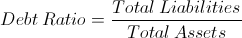# Debt RatioThe formula for the debt ratio is total liabilities divided by total assets. The debt ratio shown above is used in corporate finance and should not be confused with the debt to income ratio, sometimes shortened to debt ratio, used in consumer lending.

The debt ratio is a financial leverage ratio used along with other financial leverage ratios to measure a company's ability to handle its obligations. If a company is overleveraged, i.e. has too much debt, they may find it difficult to maintain their solvency and/or acquire new debt. Just as in consumer loans, companies are evaluated when taking on new obligations to determine their risk of non-repayment.

Both the total liabilities and total assets can be found on a company's balance sheet.

## Use of the Debt Ratio Formula

The debt ratio formula can be used by a company internally and also can be used by investors and debtors. Each financial analysis formula in isolation is not all too important as surveying the entire landscape. For example, how much of the total liabilities is long term versus current liabilities? The current ratio can be used in lieu of the debt ratio formula to gauge short term solvency.

As exampled above, the debt ratio formula is but one aspect of a company's financial story. The plot thickens as the story evolves. The debt ratio individually shows a macro-level view of a company's debt load relative to the assets of the company.

## Example of the Debt Ratio Formula

A simple example of the debt ratio formula would be a company who has total assets of \$3 million and total liabilities of \$2.5 million. The total liabilities of \$2.5 million would be divided by the total assets of \$3 million which gives a debt ratio of .8333.

New to Finance?